This documentation is for scikit-learn version 0.16.1Other versions

If you use the software, please consider citing scikit-learn.

# 3.1. Cross-validation: evaluating estimator performance¶

Learning the parameters of a prediction function and testing it on the same data is a methodological mistake: a model that would just repeat the labels of the samples that it has just seen would have a perfect score but would fail to predict anything useful on yet-unseen data. This situation is called overfitting. To avoid it, it is common practice when performing a (supervised) machine learning experiment to hold out part of the available data as a test set X_test, y_test. Note that the word “experiment” is not intended to denote academic use only, because even in commercial settings machine learning usually starts out experimentally.

In scikit-learn a random split into training and test sets can be quickly computed with the train_test_split helper function. Let’s load the iris data set to fit a linear support vector machine on it:

```>>> import numpy as np
>>> from sklearn import cross_validation
>>> from sklearn import datasets
>>> from sklearn import svm

>>> iris.data.shape, iris.target.shape
((150, 4), (150,))
```

We can now quickly sample a training set while holding out 40% of the data for testing (evaluating) our classifier:

```>>> X_train, X_test, y_train, y_test = cross_validation.train_test_split(
...     iris.data, iris.target, test_size=0.4, random_state=0)

>>> X_train.shape, y_train.shape
((90, 4), (90,))
>>> X_test.shape, y_test.shape
((60, 4), (60,))

>>> clf = svm.SVC(kernel='linear', C=1).fit(X_train, y_train)
>>> clf.score(X_test, y_test)
0.96...
```

When evaluating different settings (“hyperparameters”) for estimators, such as the C setting that must be manually set for an SVM, there is still a risk of overfitting on the test set because the parameters can be tweaked until the estimator performs optimally. This way, knowledge about the test set can “leak” into the model and evaluation metrics no longer report on generalization performance. To solve this problem, yet another part of the dataset can be held out as a so-called “validation set”: training proceeds on the training set, after which evaluation is done on the validation set, and when the experiment seems to be successful, final evaluation can be done on the test set.

However, by partitioning the available data into three sets, we drastically reduce the number of samples which can be used for learning the model, and the results can depend on a particular random choice for the pair of (train, validation) sets.

A solution to this problem is a procedure called cross-validation (CV for short). A test set should still be held out for final evaluation, but the validation set is no longer needed when doing CV. In the basic approach, called k-fold CV, the training set is split into k smaller sets (other approaches are described below, but generally follow the same principles). The following procedure is followed for each of the k “folds”:

• A model is trained using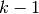of the folds as training data;
• the resulting model is validated on the remaining part of the data (i.e., it is used as a test set to compute a performance measure such as accuracy).

The performance measure reported by k-fold cross-validation is then the average of the values computed in the loop. This approach can be computationally expensive, but does not waste too much data (as it is the case when fixing an arbitrary test set), which is a major advantage in problem such as inverse inference where the number of samples is very small.

## 3.1.1. Computing cross-validated metrics¶

The simplest way to use cross-validation is to call the cross_val_score helper function on the estimator and the dataset.

The following example demonstrates how to estimate the accuracy of a linear kernel support vector machine on the iris dataset by splitting the data, fitting a model and computing the score 5 consecutive times (with different splits each time):

```>>> clf = svm.SVC(kernel='linear', C=1)
>>> scores = cross_validation.cross_val_score(
...    clf, iris.data, iris.target, cv=5)
...
>>> scores
array([ 0.96...,  1.  ...,  0.96...,  0.96...,  1.        ])
```

The mean score and the 95% confidence interval of the score estimate are hence given by:

```>>> print("Accuracy: %0.2f (+/- %0.2f)" % (scores.mean(), scores.std() * 2))
Accuracy: 0.98 (+/- 0.03)
```

By default, the score computed at each CV iteration is the score method of the estimator. It is possible to change this by using the scoring parameter:

```>>> from sklearn import metrics
>>> scores = cross_validation.cross_val_score(clf, iris.data, iris.target,
...     cv=5, scoring='f1_weighted')
>>> scores
array([ 0.96...,  1.  ...,  0.96...,  0.96...,  1.        ])
```

See The scoring parameter: defining model evaluation rules for details. In the case of the Iris dataset, the samples are balanced across target classes hence the accuracy and the F1-score are almost equal.

When the cv argument is an integer, cross_val_score uses the KFold or StratifiedKFold strategies by default, the latter being used if the estimator derives from ClassifierMixin.

It is also possible to use other cross validation strategies by passing a cross validation iterator instead, for instance:

```>>> n_samples = iris.data.shape
>>> cv = cross_validation.ShuffleSplit(n_samples, n_iter=3,
...     test_size=0.3, random_state=0)

>>> cross_validation.cross_val_score(clf, iris.data, iris.target, cv=cv)
...
array([ 0.97...,  0.97...,  1.        ])
```

Data transformation with held out data

Just as it is important to test a predictor on data held-out from training, preprocessing (such as standardization, feature selection, etc.) and similar data transformations similarly should be learnt from a training set and applied to held-out data for prediction:

```>>> from sklearn import preprocessing
>>> X_train, X_test, y_train, y_test = cross_validation.train_test_split(
...     iris.data, iris.target, test_size=0.4, random_state=0)
>>> scaler = preprocessing.StandardScaler().fit(X_train)
>>> X_train_transformed = scaler.transform(X_train)
>>> clf = svm.SVC(C=1).fit(X_train_transformed, y_train)
>>> X_test_transformed = scaler.transform(X_test)
>>> clf.score(X_test_transformed, y_test)
0.9333...
```

A Pipeline makes it easier to compose estimators, providing this behavior under cross-validation:

```>>> from sklearn.pipeline import make_pipeline
>>> clf = make_pipeline(preprocessing.StandardScaler(), svm.SVC(C=1))
>>> cross_validation.cross_val_score(clf, iris.data, iris.target, cv=cv)
...
array([ 0.97...,  0.93...,  0.95...])
```

### 3.1.1.1. Obtaining predictions by cross-validation¶

The function cross_val_predict has a similar interface to cross_val_score, but returns, for each element in the input, the prediction that was obtained for that element when it was in the test set. Only cross-validation strategies that assign all elements to a test set exactly once can be used (otherwise, an exception is raised).

These prediction can then be used to evaluate the classifier:

```>>> predicted = cross_validation.cross_val_predict(clf, iris.data,
...                                                iris.target, cv=10)
>>> metrics.accuracy_score(iris.target, predicted)
0.966...
```

Note that the result of this computation may be slightly different from those obtained using cross_val_score as the elements are grouped in different ways.

The available cross validation iterators are introduced in the following section.

## 3.1.2. Cross validation iterators¶

The following sections list utilities to generate indices that can be used to generate dataset splits according to different cross validation strategies.

### 3.1.2.1. K-fold¶

KFold divides all the samples in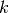groups of samples, called folds (if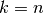, this is equivalent to the Leave One Out strategy), of equal sizes (if possible). The prediction function is learned usingfolds, and the fold left out is used for test.

Example of 2-fold cross-validation on a dataset with 4 samples:

```>>> import numpy as np
>>> from sklearn.cross_validation import KFold

>>> kf = KFold(4, n_folds=2)
>>> for train, test in kf:
...     print("%s %s" % (train, test))
[2 3] [0 1]
[0 1] [2 3]
```

Each fold is constituted by two arrays: the first one is related to the training set, and the second one to the test set. Thus, one can create the training/test sets using numpy indexing:

```>>> X = np.array([[0., 0.], [1., 1.], [-1., -1.], [2., 2.]])
>>> y = np.array([0, 1, 0, 1])
>>> X_train, X_test, y_train, y_test = X[train], X[test], y[train], y[test]
```

### 3.1.2.2. Stratified k-fold¶

StratifiedKFold is a variation of k-fold which returns stratified folds: each set contains approximately the same percentage of samples of each target class as the complete set.

Example of stratified 2-fold cross-validation on a dataset with 10 samples from two slightly unbalanced classes:

```>>> from sklearn.cross_validation import StratifiedKFold

>>> labels = [0, 0, 0, 0, 1, 1, 1, 1, 1, 1]
>>> skf = StratifiedKFold(labels, 3)
>>> for train, test in skf:
...     print("%s %s" % (train, test))
[2 3 6 7 8 9] [0 1 4 5]
[0 1 3 4 5 8 9] [2 6 7]
[0 1 2 4 5 6 7] [3 8 9]
```

### 3.1.2.3. Leave-One-Out - LOO¶

LeaveOneOut (or LOO) is a simple cross-validation. Each learning set is created by taking all the samples except one, the test set being the sample left out. Thus, for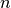samples, we havedifferent training sets anddifferent tests set. This cross-validation procedure does not waste much data as only one sample is removed from the training set:

```>>> from sklearn.cross_validation import LeaveOneOut

>>> loo = LeaveOneOut(4)
>>> for train, test in loo:
...     print("%s %s" % (train, test))
[1 2 3] 
[0 2 3] 
[0 1 3] 
[0 1 2] 
```

Potential users of LOO for model selection should weigh a few known caveats. When compared with-fold cross validation, one buildsmodels fromsamples instead ofmodels, where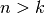. Moreover, each is trained on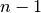samples rather than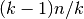. In both ways, assumingis not too large and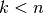, LOO is more computationally expensive than-fold cross validation.

In terms of accuracy, LOO often results in high variance as an estimator for the test error. Intuitively, sinceof thesamples are used to build each model, models constructed from folds are virtually identical to each other and to the model built from the entire training set.

However, if the learning curve is steep for the training size in question, then 5- or 10- fold cross validation can overestimate the generalization error.

As a general rule, most authors, and empirical evidence, suggest that 5- or 10- fold cross validation should be preferred to LOO.

References:

### 3.1.2.4. Leave-P-Out - LPO¶

LeavePOut is very similar to LeaveOneOut as it creates all the possible training/test sets by removing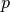samples from the complete set. Forsamples, this produces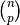train-test pairs. Unlike LeaveOneOut and KFold, the test sets will overlap for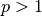.

Example of Leave-2-Out on a dataset with 4 samples:

```>>> from sklearn.cross_validation import LeavePOut

>>> lpo = LeavePOut(4, p=2)
>>> for train, test in lpo:
...     print("%s %s" % (train, test))
[2 3] [0 1]
[1 3] [0 2]
[1 2] [0 3]
[0 3] [1 2]
[0 2] [1 3]
[0 1] [2 3]
```

### 3.1.2.5. Leave-One-Label-Out - LOLO¶

LeaveOneLabelOut (LOLO) is a cross-validation scheme which holds out the samples according to a third-party provided array of integer labels. This label information can be used to encode arbitrary domain specific pre-defined cross-validation folds.

Each training set is thus constituted by all the samples except the ones related to a specific label.

For example, in the cases of multiple experiments, LOLO can be used to create a cross-validation based on the different experiments: we create a training set using the samples of all the experiments except one:

```>>> from sklearn.cross_validation import LeaveOneLabelOut

>>> labels = [1, 1, 2, 2]
>>> lolo = LeaveOneLabelOut(labels)
>>> for train, test in lolo:
...     print("%s %s" % (train, test))
[2 3] [0 1]
[0 1] [2 3]
```

Another common application is to use time information: for instance the labels could be the year of collection of the samples and thus allow for cross-validation against time-based splits.

Warning

Contrary to StratifiedKFold, the ``labels`` of :class:`LeaveOneLabelOut` should not encode the target class to predict: the goal of StratifiedKFold is to rebalance dataset classes across the train / test split to ensure that the train and test folds have approximately the same percentage of samples of each class while LeaveOneLabelOut will do the opposite by ensuring that the samples of the train and test fold will not share the same label value.

### 3.1.2.6. Leave-P-Label-Out¶

LeavePLabelOut is similar as Leave-One-Label-Out, but removes samples related to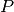labels for each training/test set.

Example of Leave-2-Label Out:

```>>> from sklearn.cross_validation import LeavePLabelOut

>>> labels = [1, 1, 2, 2, 3, 3]
>>> lplo = LeavePLabelOut(labels, p=2)
>>> for train, test in lplo:
...     print("%s %s" % (train, test))
[4 5] [0 1 2 3]
[2 3] [0 1 4 5]
[0 1] [2 3 4 5]
```

### 3.1.2.7. Random permutations cross-validation a.k.a. Shuffle & Split¶

ShuffleSplit

The ShuffleSplit iterator will generate a user defined number of independent train / test dataset splits. Samples are first shuffled and then split into a pair of train and test sets.

It is possible to control the randomness for reproducibility of the results by explicitly seeding the random_state pseudo random number generator.

Here is a usage example:

```>>> ss = cross_validation.ShuffleSplit(5, n_iter=3, test_size=0.25,
...     random_state=0)
>>> for train_index, test_index in ss:
...     print("%s %s" % (train_index, test_index))
...
[1 3 4] [2 0]
[1 4 3] [0 2]
[4 0 2] [1 3]
```

ShuffleSplit is thus a good alternative to KFold cross validation that allows a finer control on the number of iterations and the proportion of samples in on each side of the train / test split.

### 3.1.2.8. Predefined Fold-Splits / Validation-Sets¶

For some datasets, a pre-defined split of the data into training- and validation fold or into several cross-validation folds already exists. Using PredefinedSplit it is possible to use these folds e.g. when searching for hyperparameters.

For example, when using a validation set, set the test_fold to 0 for all samples that are part of the validation set, and to -1 for all other samples.

StratifiedShuffleSplit is a variation of ShuffleSplit, which returns stratified splits, i.e which creates splits by preserving the same percentage for each target class as in the complete set.

## 3.1.3. A note on shuffling¶

If the data ordering is not arbitrary (e.g. samples with the same label are contiguous), shuffling it first may be essential to get a meaningful cross- validation result. However, the opposite may be true if the samples are not independently and identically distributed. For example, if samples correspond to news articles, and are ordered by their time of publication, then shuffling the data will likely lead to a model that is overfit and an inflated validation score: it will be tested on samples that are artificially similar (close in time) to training samples.

Some cross validation iterators, such as KFold, have an inbuilt option to shuffle the data indices before splitting them. Note that:

• This consumes less memory than shuffling the data directly.
• By default no shuffling occurs, including for the (stratified) K fold cross- validation performed by specifying cv=some_integer to cross_val_score, grid search, etc. Keep in mind that train_test_split still returns a random split.
• The random_state parameter defaults to None, meaning that the shuffling will be different every time KFold(..., shuffle=True) is iterated. However, GridSearchCV will use the same shuffling for each set of parameters validated by a single call to its fit method.
• To ensure results are repeatable (on the same platform), use a fixed value for random_state.

## 3.1.4. Cross validation and model selection¶

Cross validation iterators can also be used to directly perform model selection using Grid Search for the optimal hyperparameters of the model. This is the topic if the next section: Grid Search: Searching for estimator parameters.